Tutorials
python
+2

Introduction to Deep Learning in Python

Learn the basics of deep learning and neural networks along with some fundamental concepts and terminologies used in deep learning.

In this post, you will be introduced to the magical world of deep learning. This tutorial will mostly cover the basics of deep learning and neural networks. You will learn some fundamental concepts and terminologies used in deep learning, and understand why deep learning techniques are so powerful today. Not only that, but you will also build a simple neural network all by yourself and generate predictions using python's numpy library.

Tip: For a little background on Artificial Intelligence, Machine Learning & Deep Learning consider reading a post on Differences Between Machine Learning & Deep Learning or for an introduction on machine learning this short tutorial might be helpful.

Introduction

Imagine you work for a loan company, and you need to build a model for predicting, whether a user (borrower) should get a loan or not? You have the features for each customer like age, bank balance, salary per annum, whether retired or not and so on.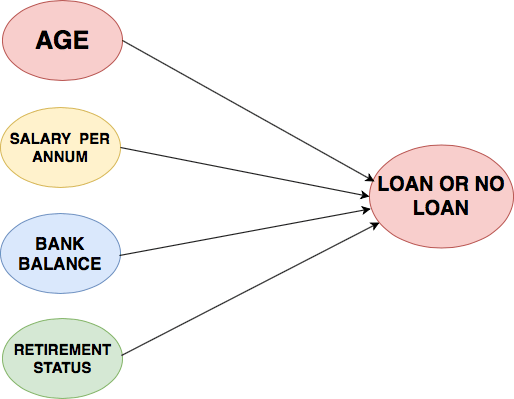Consider if you want to solve this problem using a linear regression model, then the linear regression will assume that the outcome (whether a customer's loan should be sanctioned or not) will be the sum of all the features. It will take into account the effect of age, salary, bank balance, retirement status and so. So the linear regression model is not taking into account the interaction between these features or how they affect the overall loan process.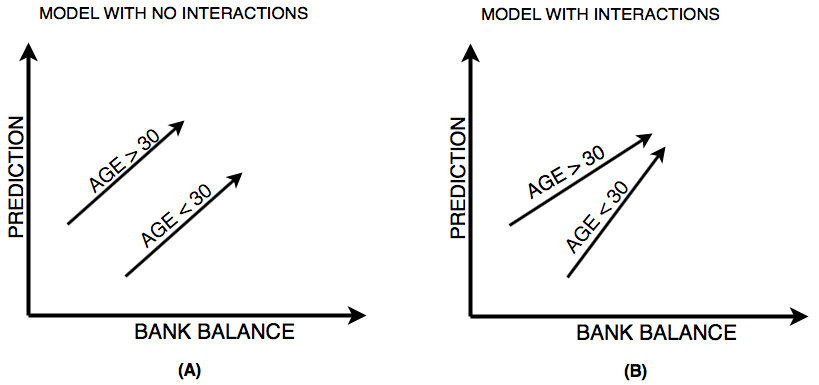The above figure left (A) shows prediction from a linear regression model with absolutely no interactions in which it simply adds up the effect of age (30 > age > 30) and bank balance, you can observe from figure (A) that the lack of interaction is reflected by both lines being parallel that is what the linear regression model assumes.

On the other hand, figure right (B) shows predictions from a model that allows interactions in which the lines do not have to parallel. Neural Networks is a pretty good modeling approach that allows interactions like the one in figure (B) very well and from these neural networks evolves a term known as Deep Learning which uses these powerful neural networks. Because the neural network takes into account these type of interactions so well it can perform quite well on a plethora of prediction problems you have seen till now or possibly not heard.

Since neural networks are capable of handling such complex interactions gives them the power to solve challenging problems and do amazing things with

• Image
• Text
• Audio
• Video

This list is merely a subset of what neural networks are capable of solving, almost anything you can think of in data science field can be solved with neural networks.

Deep learning can even learn to write a code for you. Well isn't that super amazing?

Interactions in Neural Network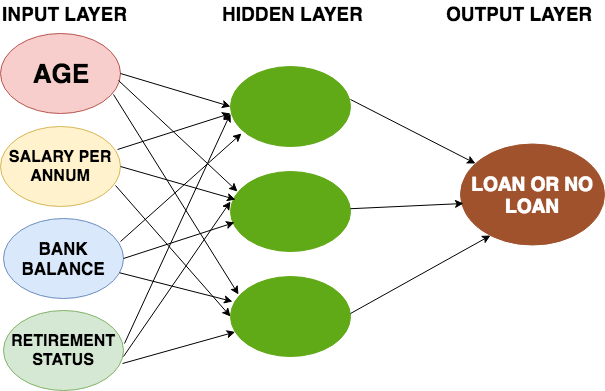The neural network architecture looks something similar to the above figure. On the far left you have the input layer that consists of the features like age, salary per annum, bank balance, etc. and on the far right, you have the output layer that outputs the prediction from the model which in your case is whether a customer should get a loan or not.

The layers apart from the input and the output layers are called the hidden layers.

Now the question is why they are called hidden layers?

Well, one good reason is while the input and output layers correspond to apparent things that occur or are present in the world and can be stored as data but the values in the hidden layers are not something that relates to the real world or something for which have data.

Technically, each node in the hidden layer represents an aggregation of information from the input data; hence each node adds to the model's capability to capture interactions between the data. The more the nodes, the more interactions can be achieved from the data.

Forward Propagation

Let's start by seeing how neural networks use data to make predictions which is taken care by the forward propagation algorithm.

To understand the concept of forward propagation let's revisit the example of a loan company. For simplification, let's consider only two features as an input namely age and retirement status, the retirement status being a binary ( 0 - not retired and 1 - retired) number based on which you will make predictions.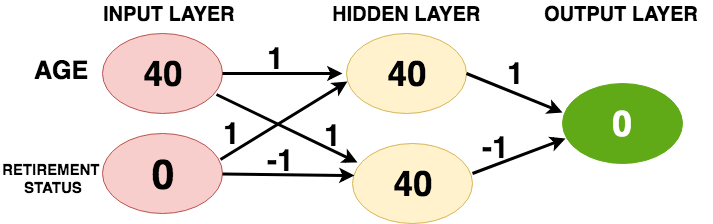The above figure shows a customer with age 40 and is not retired. The forward propagation algorithm will pass this information through the network/model to predict the output layer. The lines connect each node of the input to every other node of the hidden layer. Each line has a weight associated with it which indicates how strongly that feature affects the hidden node connected to that specific line.

There are total four weights between input and hidden layer. The first set of weights are connected from the top node of the input layer to the first and second node of the hidden layer; likewise, the second set of weight are connected from the bottom node of the input to the first and second node of the hidden layer.

Remember these weights are the key in deep learning which you train or update when you fit a neural network to the data. These weights are commonly known as parameters.

To make a prediction for the top node of the hidden layer, you consider each node in the input layer multiply it by the weights connected to that top node and finally sum up all the values resulting in a value 40 (40 * 1 + 0 * 1 = 40) as shown in above figure. You repeat the same process for the bottom node of the hidden layer resulting in a value 40. Finally, for the output layer you follow the same process and obtain a value 0 (40 * 1 + 40 * (-1) = 0). This output layer predicts a value zero.

Now you might wonder what the relevance of value zero is, well you consider the loan problem as binary classification in which an output of zero indicates a loan sanction and an output of one indicates a loan prohibition.

That's pretty much what happens in forward propagation. You start from the input layer move to the hidden layer and then to the output layer which then gives you a prediction score. You pretty much always use the multiple-add process, in linear algebra this operation is a dot product operation. In general, a forward propagation is done for a single data point at a time.

It's time to see the code for the above forward propagation algorithm!

To do this, you will first import a great python library called numpy.

import numpy as np

Next, you will create a numpy array of input_data.

input_data = np.array([40,0])

Once you have the input data, now you will create a dictionary called weights in which the keys of the dictionary will hold the variable names for node0 and node1 of hidden layers and an output node for the output layer. The values of the dictionary will be the parameters (weight values).

weights = {'node0':([1,1]),
'node1':([1,-1]),
'output':([1,-1])}

Let's quickly calculate the value of node0 of the hidden layer. You first multiply the input_data with the weights of node0 and then use a sum() function to obtain a scalar value.

node0_value = (input_data * weights['node0']).sum()

You will do the same for the node1 of the hidden layer.

node1_value = (input_data * weights['node1']).sum()

For simplicity let's create a numpy array of the hidden layer values.

hidden_layer_values = np.array([node0_value,node1_value])
hidden_layer_values
array([40, 40])

Finally, you multiply the hidden layer values with the weights of the output layer and again use a sum() function to obtain a prediction.

output = (hidden_layer_values * weights['output']).sum()

Let's print the output and see if it matches the output you should expect!

output
0

Activation Functions

The multiply-add process is only half part of how a neural network works; there's more to it!

To utilize the maximum predictive power, a neural network uses an activation function in the hidden layers. An activation function allows the neural network to capture non-linearities present in the data.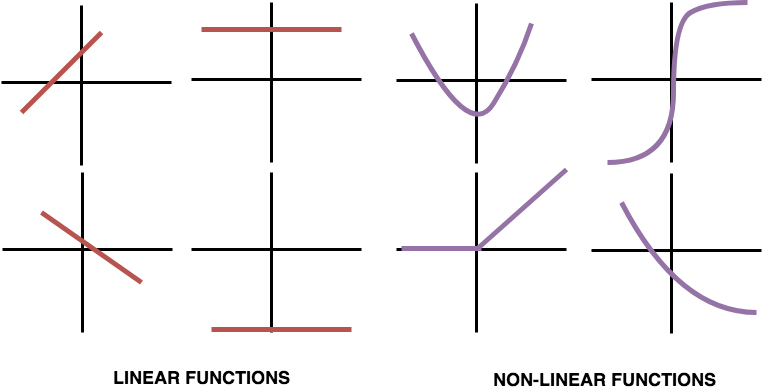In neural networks, often time the data that you work with is not linearly separable and to find a decision boundary that can separate the data points you need some non-linearity in your network. For example, A customer has no previous loan record compared to a customer having a previous loan record may impact the overall output differently.

If the relationships in the data aren't straight or linear, then you need a non-linear activation function to capture the non-linearity. An activation function is applied to the value coming into a node which then transforms it into the value stored in that node or the node output.

Let's apply an s-shaped activation function called tanh to the nodes of hidden layers.

node0_act = np.tanh(node0_value)
node1_act = np.tanh(node1_value)
hidden_layer_values_act = np.array([node0_act,node1_act])
hidden_layer_values_act
array([1., 1.])

You can observe the difference in the hidden_layer_values and hidden_layer_values_act.

Let's quickly calculate the output using the hidden_layer_values_act.

output = (hidden_layer_values_act * weights['output']).sum()
output
0.0

In today's time, an activation function called Rectifier Linear Unit (ReLU) is widely used in both industry and research. Even though it has two linear pieces, it's very powerful when combined through multiple hidden layers. ReLU is half rectified from the bottom as shown in the figure below.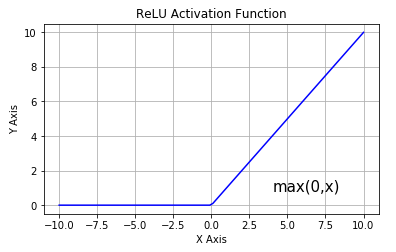(Source)

Now you will apply ReLU as the activation function on the hidden layer nodes and calculate the network's output.

def relu(input):
'''Define your relu activation function here'''
# Calculate the value for the output of the relu function: output
output = max(0, input)

# Return the value just calculated
return(output)
# Calculate node 0 value: node_0_output
node_0_input = (input_data * weights['node0']).sum()
node_0_output = relu(node_0_input)

# Calculate node 1 value: node_1_output
node_1_input = (input_data * weights['node1']).sum()
node_1_output = relu(node_1_input)

# Put node values into array: hidden_layer_outputs
hidden_layer_outputs = np.array([node_0_output, node_1_output])

# Calculate model output (do not apply relu)
model_output = (hidden_layer_outputs * weights['output']).sum()

# Print model output
print("Model's Output:",model_output)
Model's Output: 0

Similar to the above example wherein you had just one data point (observation), you can implement the same network for multiple observations or rows of data.

Deep Learning (Deeper Networks)

The significant difference between traditional neural networks and the modern deep learning that makes use of neural networks is the use of not just one but many successive hidden layers. Research shows that increasing the number of hidden layers massively improves the performance making the network capable of more and more interactions.

The working in a network with just a single hidden layer and with multiple hidden layers remain the same. You forward propagate through these successive hidden layers as you did in the previous example with one hidden layer.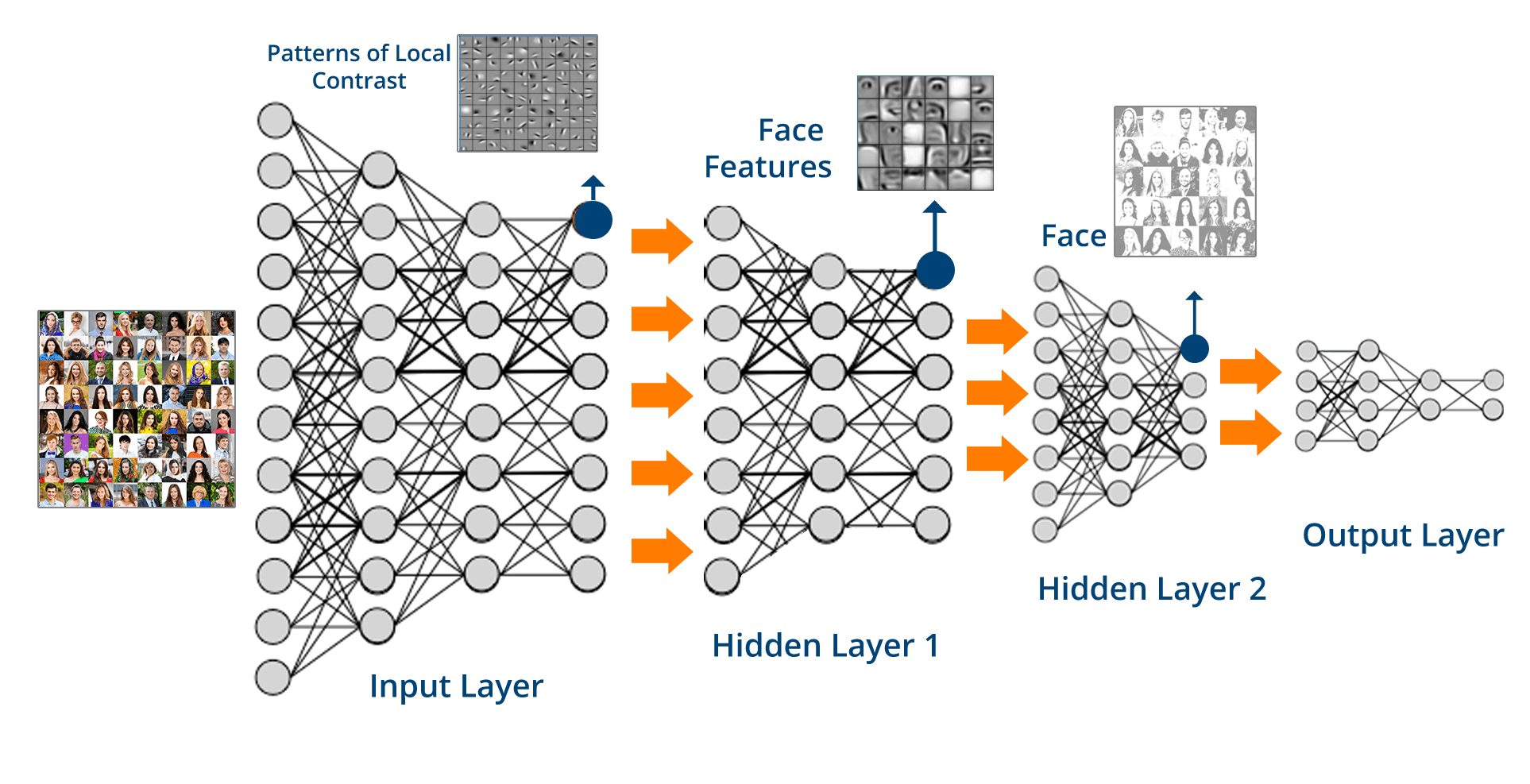(Source)

Let's understand some essential facts about these deep networks!

• Deep Learning networks are capable of internally building up representations of the pattern in the data that are essential for making accurate predictions;
• The patterns in the initial layers are simple, but as you go through successive hidden layers or deep into the network the network starts learning more and more complex patterns;
• Deep learning networks eliminate the need for handcrafted features. You do not need to create better predictive features which you then feed to the deep learning network, the network itself learns meaningful features from the data and using which it makes predictions;
• Deep learning is also called Representation Learning since the subsequent layers in the network build increasingly sophisticated representations of the data until you reach to the final layer where it finally makes the prediction.

Let's try to get some understanding about the representations that the network tries to capture, from the above figure.

The input to the above network is images of humans; You can see that the initial layers in the network are capturing the patterns of local contrast that are conceptually simple, patterns like vertical edges, horizontal, diagonal edges, blurry areas, etc. Once the network identifies where are these diagonal or horizontal lines the successive layers then combine that information to find larger patterns like eyes, nose, lips, etc. A much later layer might combine these patterns to find much larger abstract patterns like for example a face as depicted in the above figure.

Well, the cool thing about deep learning is you don't explicitly tell the network to look for diagonal lines or wherein the image is the nose or a lip, instead of when you train the network the neural network has weights that are learned to find the relevant patterns to make accurate predictions. The learning process in neural networks off course is a gradual process in which the network undergoes multiple pieces of training before it can learn to make better predictions.

Forward propagation in a multi-layer neural network

input_data = np.array([3,5])
weights = {'node0_0':([2,4]),
'node0_1':([4,-5]),
'node1_0':([-1,2]),
'node1_1':([1,2]),
'output':([2,7])}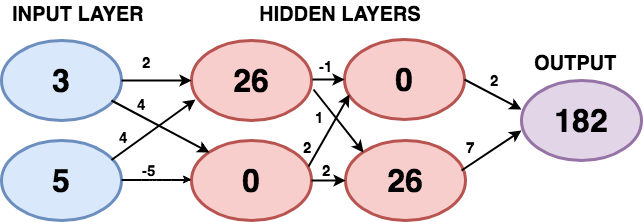def predict_with_network(input_data):
# Calculate node 0 in the first hidden layer
node_0_0_input = (input_data* weights['node0_0']).sum()
node_0_0_output = relu(node_0_0_input)

# Calculate node 1 in the first hidden layer
node_0_1_input = (input_data* weights['node0_1']).sum()
node_0_1_output = relu(node_0_1_input)

# Put node values into array: hidden_0_outputs
hidden_0_outputs = np.array([node_0_0_output, node_0_1_output])
print("Hidden Layer 1 Output:", hidden_0_outputs)

# Calculate node 0 in the second hidden layer
node_1_0_input =  (hidden_0_outputs* weights['node1_0']).sum()
node_1_0_output = relu(node_1_0_input)

# Calculate node 1 in the second hidden layer
node_1_1_input = (hidden_0_outputs* weights['node1_1']).sum()
node_1_1_output = relu(node_1_1_input)

# Put node values into array: hidden_1_outputs
hidden_1_outputs = np.array([node_1_0_output, node_1_1_output])
print("Hidden Layer 2 Output:", hidden_1_outputs)
# Calculate model output: model_output
model_output = (hidden_1_outputs * weights['output']).sum()
# Return model_output
return(model_output)

output = predict_with_network(input_data)
print("Model's Prediction:",output)
Hidden Layer 1 Output: [26  0]
Hidden Layer 2 Output: [ 0 26]
Model's Prediction: 182

Tip: If you were able to understand till here then you must consider reading a post on Convolutional Neural Networks in Python with Keras which teaches you a new terminology known as convolutional neural networks, not only that it also uses a deep learning framework called Keras along with a famous image dataset to classify handwritten digits and a lot more.

Go Further!

Congratulations to all of you who successfully made it till the end!

This post tried to give you an intuitive understanding about the powerful neural networks, how the neural network learns using forward propagation algorithm and how you can implement forward propagation using a python's library called numpy with some facts on the deep neural networks.

The learning in neural networks has more components attached to it apart from a forward propagation algorithm so to dive deeper into neural networks and how you can make predictions using this magical network consider taking up a course on Deep Learning in Python. This course will also teach you a deep learning framework called Keras using which in just a few lines of code you can train very deep neural networks.